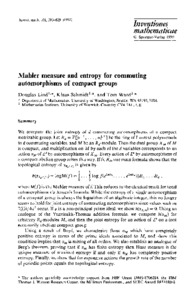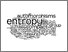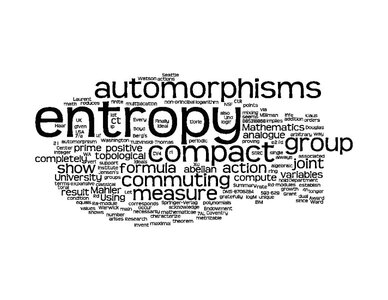# Mahler measure and entropy for commuting automorphisms of compact groups

Lind, Douglas, Schmidt, Klaus and Ward, Tom (1990) Mahler measure and entropy for commuting automorphisms of compact groups. Inventiones Mathematicae, 101 (1). pp. 593-629. ISSN 0020-9910Preview PDF (mahlerentropy.pdf) - Published Version Download (1MB) | PreviewPreview Image (JPEG) (2.jpg) Download (80kB) | Preview

## Abstract

We compute the joint entropy of d commuting automorphisms of a compact metrizable group. Let Rd = Z[u1±1,...,ud±1] be the ring of Laurent polynomials in d commuting variables, and let M be an Rd-module. Then the dual group XM of M is compact, and multiplication by each of the d variables corresponds to an action aM of Zd by automorphisms of XM. Every action of Zd by automorphisms of a compact abelian group arises this way. If f Î Rd, our main formula shows that the topological entropy of aRd/áfñ > is given by where M(f) is the Mahler measure of f. This reduces to the classical result for toral automorphisms via Jensen's formula. While the entropy of a single automorphism of a compact group is always the logarithm of an algebraic integer, this no longer seems to hold for joint entropy of commuting automorphisms since values such as 7z(3)/4p2 occur. If p is a non-principal prime ideal, we show h(aRd/p) = 0. Using an analogue of the Yuzvinskii-Thomas addition formula, we compute h(aM) for arbitrary Rd-modules M, and then the joint entropy for an action of Zd on a (not necessarily abelian) compact group. Using a result of Boyd, we characterize those aM which have completely positive entropy in terms of the prime ideals associated to M, and show this condition implies that aM is mixing of all orders. We also establish an analogue of Berg's theorem, proving that if aM has finite entropy then Haar measure is the unique measure of maximal entropy if and only if aM has completely positive entropy. Finally, we show that for expansive actions the growth rate of the number of periodic points equals the topological entropy.

Item Type: Article Faculty of Science > School of Mathematics Vishal Gautam 18 Mar 2011 14:48 15 Dec 2022 02:11 https://ueaeprints.uea.ac.uk/id/eprint/18590 10.1007/BF01231517View Item